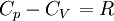# Difference between revisions of "Ideal gas: Heat capacity"

Jump to: navigation, search$C_p - C_V = \left.\frac{\partial V}{\partial T}\right\vert_p \left(p + \left.\frac{\partial E}{\partial V}\right\vert_T \right)$$\left.C_p -C_V \right.=R$